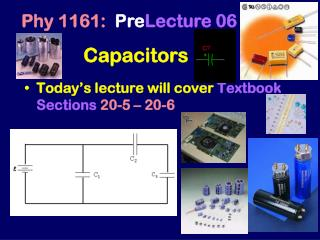DownloadDownload PresentationCapacitors

# Capacitors

Download Presentation## Capacitors

- - - - - - - - - - - - - - - - - - - - - - - - - - - E N D - - - - - - - - - - - - - - - - - - - - - - - - - - -
##### Presentation Transcript

1. Phy 1161: PreLecture 06 Capacitors • Today’s lecture will coverTextbook Sections20-5 – 20-6

2. q A B Comparison:Electric Potential Energy vs. Electric Potential DVAB: the difference in electric potential between points B and A DUAB : the change in electric potential energy of a charge q when moved from A to B DUAB = q DVAB

3. Electric Potential: Summary • E field lines point from higher to lower potential • For positive charges, going from higher to lower potential is “downhill” • For a battery, the (+) terminal is at a higher potential than the (–) terminal Positive charges tend to go “downhill”, from + to - Negative charges go in the opposite direction, from - to + DUAB = q DVAB

4. Important Special CaseUniform Electric Field - - - - - + + + + + • Two large parallel conducting plates of area A • +Q on one plate • -Q on other plate • Then E is • uniform between the two plates: E=4kQ/A • zero everywhere else • This result is independent of plate separation • This is call a parallel plate capacitor

5. A B Parallel Plate CapacitorPotential Difference Charge Q on plates Charge 2Q on plates V = VA – VB = +E0 d V = VA – VB = +2E0d E= E=E0 - - - - - + + + + + - - - - - + + + + + - - - - - + + + + + A B d d • Potential difference is proportional to charge: Double Q  Double V • E0 = 4πkQ/A

6. Capacitance • The ability to store separated charge • Definition: • Units: Farad (F) – named in honor of Michael Faraday • 1 F = 1C/V From Faraday’s notebook

7. - - - - - + + + + + E d Capacitor • Any pair of conductors separated by a small distance. (e.g. two metal plates) • Capacitor stores separated charge • Positive Q on one conductor, negative Q on other • Net charge is zero Q = CV • Stores Energy U = (½) Q V

8. Capacitance of Parallel Plate Capacitor V V = Ed ANDE = Q/(e0A) (Between two large plates) So: V = Qd//(e0A) Remember: CQ/V So: Equation based on geometry of capacitor + E - A A d If there is adielectric (κ>1) between plates C = κ C0 e0= 8.85x10-12 C2/Nm2

9. Dielectric constant (k > 1) Capacitance without dielectric Capacitance with dielectric Dielectric • Placing a dielectric between the plates increases the capacitance. C = k C0

10. Dielectrics

11. Vwire 1= 0 V Vwire 2= 5 V Vwire 3= 12 V Vwire 4= 15 V Voltage in Circuits • Elements are connected by wires. • Any connected region of wire has the same potential. • The potential difference across an element is the element’s “voltage.” C1 C2 C3 VC1= _____ V VC2= _____ V VC3= _____ V

12. Vwire 1= 0 V Vwire 2= 5 V Vwire 3= 12 V Vwire 4= 15 V Voltage in Circuits • Elements are connected by wires. • Any connected region of wire has the same potential. • The potential difference across an element is the element’s “voltage.” C1 C2 C3 VC1= 5 V VC2= 7 V VC3= 3 V

13. Ceq Capacitors in Parallel • Share Charge: Qeq = Q1 + Q2 • Total Cap: Ceq = (Q1 + Q2)/V = C1 + C2 • Same voltage: V1 = V2 = Veq • Both ends connected together by wire C1 C2

14. 15 V Ceq 10 V Capacitors in Parallel • Share Charge: Qeq = Q1+ Q2 • Total Cap: Ceq = (Q1+ Q2)/V = C1+ C2 • Same voltage: V1 = V2 = Veq • Both ends connected together by wire 15 V 15 V C1 C2 10 V 10 V

15. +Q -Q + + - - Ceq +Q -Q Capacitors in Series • Connected end-to-end with NO other exits • Same Charge: Q1 = Q2 = Qeq • Share Voltage: V1+V2=Veq + + + +Q + + C1 + + - + + + + C2 -Q - + - + + +

16. Electromotive Force + • Battery • Maintains potential difference V • Not constant power • Not constant current • Does NOT produce or supply charges, just “pushes” them. -